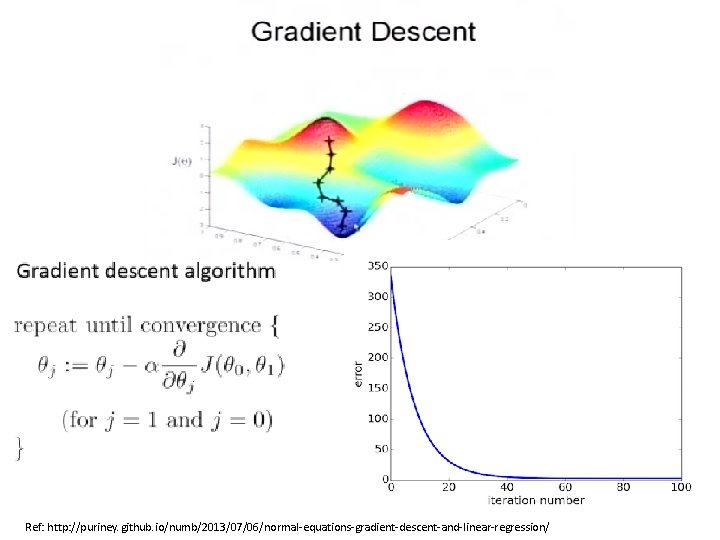# Data analysis tools Subrata Mitra and Jason Rahman

• Slides: 17Data analysis tools Subrata Mitra and Jason RahmanScikit-learn • http: //scikit-learn. org/stable/ • Python based • Tutorial is pretty good Easy to use:Python for machine learning Pros: • Python is easy to learn and use – Simplifies data preparation code • Python is a general purpose language – Integration of ML into any Python application (servers, etc) is simple • Wide variety of complementary libraries available – – – Pandas Numpy Scipy Matplotlib Seaborn Cons: • Most recent or sophisticated algorithms may not be available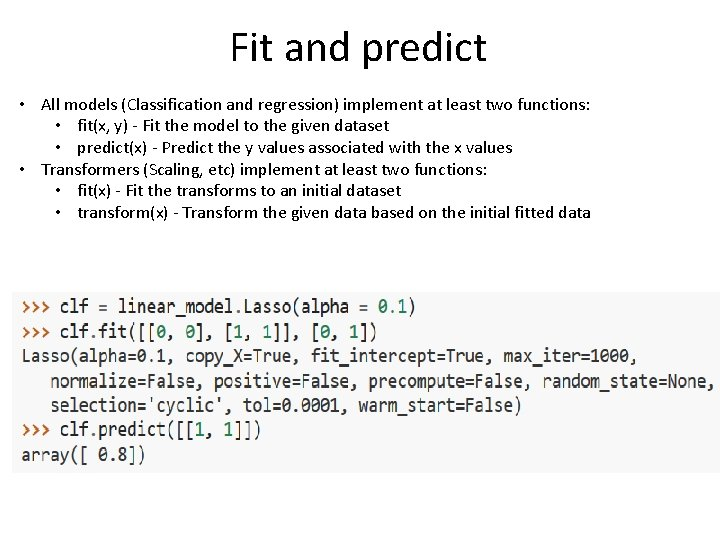Fit and predict • All models (Classification and regression) implement at least two functions: • fit(x, y) - Fit the model to the given dataset • predict(x) - Predict the y values associated with the x values • Transformers (Scaling, etc) implement at least two functions: • fit(x) - Fit the transforms to an initial dataset • transform(x) - Transform the given data based on the initial fitted dataCross validation • K-fold • Stratified k-fold: each set contains approximately the same percentage of samples of each target class as the complete set • Leave-One-Out: Each learning set is created by taking all the samples except one, the test set being the sample left out • Leave-P-Out • Shuffle & Split: Samples are first shuffled and then split into a pair of train and test sets. And many more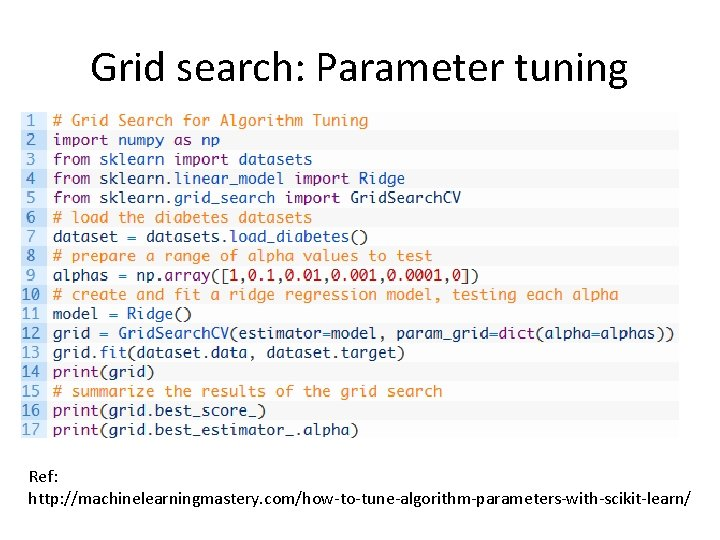Grid search: Parameter tuning Ref: http: //machinelearningmastery. com/how-to-tune-algorithm-parameters-with-scikit-learn/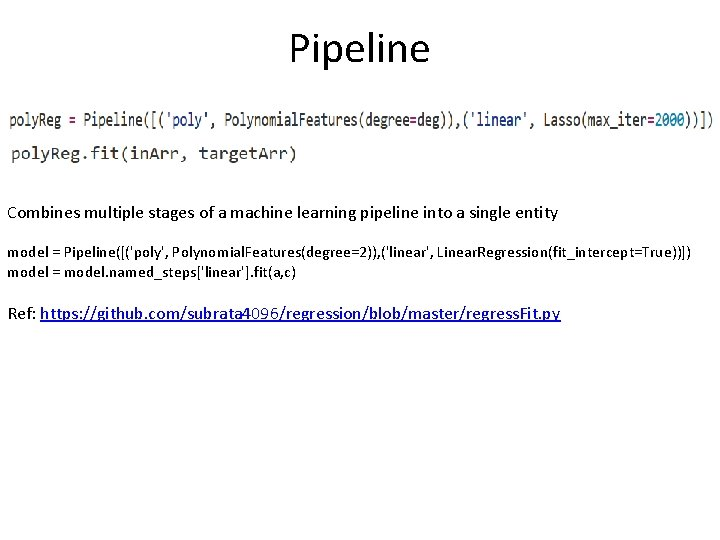Pipeline Combines multiple stages of a machine learning pipeline into a single entity model = Pipeline([('poly', Polynomial. Features(degree=2)), ('linear', Linear. Regression(fit_intercept=True))]) model = model. named_steps['linear']. fit(a, c) Ref: https: //github. com/subrata 4096/regression/blob/master/regress. Fit. py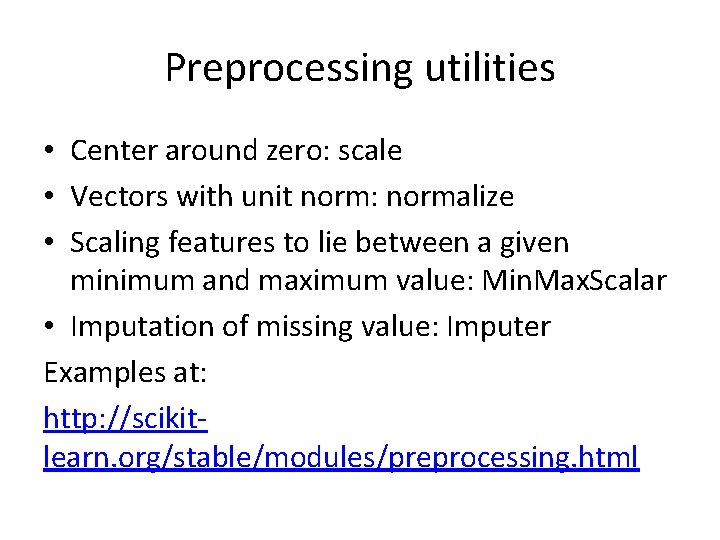Preprocessing utilities • Center around zero: scale • Vectors with unit norm: normalize • Scaling features to lie between a given minimum and maximum value: Min. Max. Scalar • Imputation of missing value: Imputer Examples at: http: //scikitlearn. org/stable/modules/preprocessing. htmlCommonly used techniques • Decision trees (Decision. Tree. Classifier, Decision. Tree. Regressor) • SVM (svm. SVC) • Regression (Linear. Regression, Ridge, Lasso, Elastic. Net) • Naive Bayes (Gaussian. NB, Multinomial. NB, Bernoulli. NB ) • All can be used with “fit”, “predict” style calls.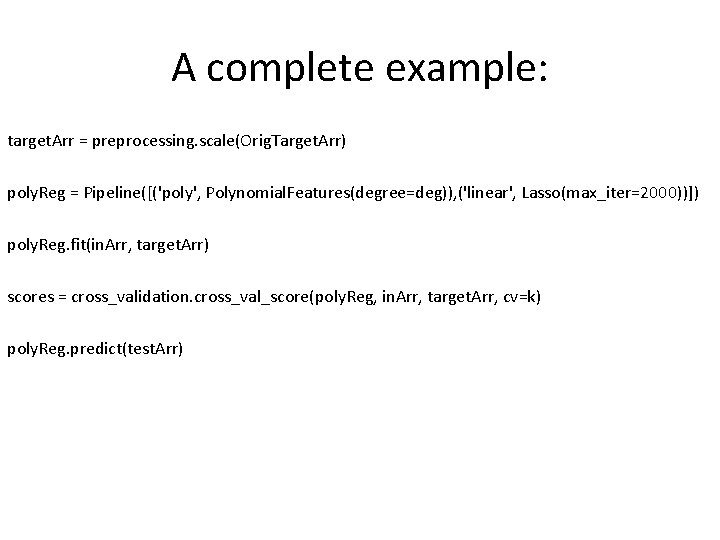A complete example: target. Arr = preprocessing. scale(Orig. Target. Arr) poly. Reg = Pipeline([('poly', Polynomial. Features(degree=deg)), ('linear', Lasso(max_iter=2000))]) poly. Reg. fit(in. Arr, target. Arr) scores = cross_validation. cross_val_score(poly. Reg, in. Arr, target. Arr, cv=k) poly. Reg. predict(test. Arr)Example: decision trees • http: //scikitlearn. org/stable/modules/tree. html#tree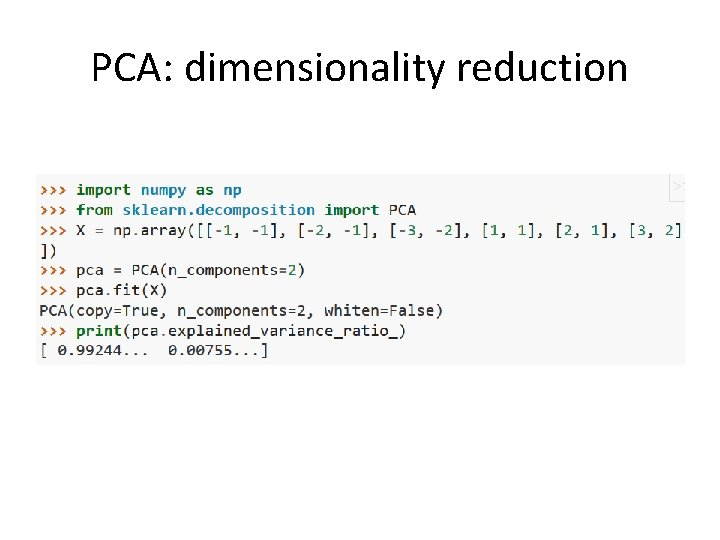PCA: dimensionality reductionOld stuffLinear regression2 -deg polynomial regression Y(w, x) = w 0 + w 1 x 1 + w 2(x 1)^2 + w 3 x 2 + w 4(x 2)^2 + w 5 x 1 x 2 Basically, you solve exactly the same way as linear regression but deal with more internally generated features.Ordinary Least Squares Ridge Regression Impose a penalty on the size of coefficients. The ridge coefficients minimize a penalized residual sum of squares. Alpha, is a complexity parameter that controls the amount of shrinkage. LASSO: Least Absolute Shrinkage & Selection Operator It is useful in some contexts due to its tendency to prefer solutions with fewer parameter values, effectively reducing the number of variables upon which the given solution is dependent.